Mathematics
Easy

Question

# Identify the first correct step for finding the sum of 23 and 8 mentally.

##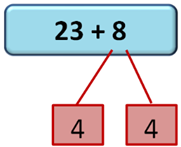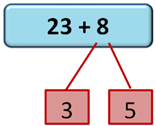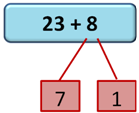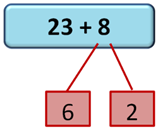Hint:

## The correct answer is:### Now as we know that here we have to find the step by breaking one number. The fact that there are no steps to memorise is one of the best things about mental math. The "friendly number" addition approach facilitates working with large numbers. This is due to the fact that we are, in essence, decomposing the problem into more manageable components.Friendly numbers are multiple of 10 like 20, 30, 40 which makes the calculations easy. Here we will use the the same and then find the step.Now we have given 23 + 8, lets solve this.We will break 8 to 7 and 1 so that it makes a friendly number, and then add 1 to it. So, 23 + 7 + 1 = 30 + 1 = 31So, the first correct step for finding the sum of 23 and 8 mentally is: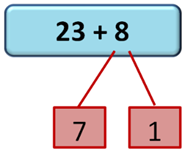Here the concept of Break Apart Numbers is used where a number is divided in two parts to make addition easy. A concept of friendly number is also used so that it becomes easy for the addition or subtraction purpose. So, the first correct step for finding the sum of 23 and 8 mentally is 23 + 7 + 1.

### Related Questions to study#### With Turito Foundation.#### Get an Expert Advice From Turito.